JAIC 1986, Volume 25, Number 1, Article 3 (pp. 31 to 37)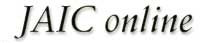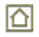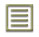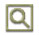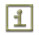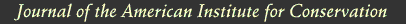JAIC 1986, Volume 25, Number 1, Article 3 (pp. 31 to 37)

### 3 RESULTS

The determination of the effective diffusivity was made by coupling Fick's First Law with the sulfur dioxide material balance over the top chamber where sulfur dioxide was entering by diffusion through the paper block. Under steady-state conditions, Fick's First Law can be assumed as: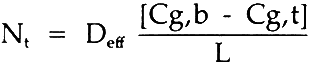where: Nt

= molar flux of sulfur dioxide through the paper;

Cg,b

= molar concentration of sulfur dioxide, bottom;

Cg,t

= molar concentration of sulfur dioxide, top;

L

= diffusion length (block thickness);

Deff

= effective diffusivity.

The sulfur dioxide material balance over the top chamber is: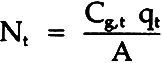where: qt

= volumetric flow rate of gas out of top chamber

A

= area of diffusion

Combining these equations gives: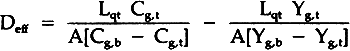Where Y represents the mole fraction or mole percent of sulfur dioxide. For this study, A = 100 cm2, L = 3.8 cm and qt and Y values were averaged for the different runs. For diffusion normal to the paper, the average diffusivity was found to be 0.0072 cm2s and for diffusion parallel to the paper, it was 0.036 cm2/s. Table 1 summarizes the experimental data used to calculate the diffusivity values.

TABLE 1 Effective Diffusivity Components of Sulfur Dioxide in Air Through Stacked Layers of Paper, Experimental Results For Diffusion Parallel to the Stacked Sheets of Paper Due to a Concentration Gradient in the Same Direction

The tortuosity of the paper block is defined by the parallel pore model equation:10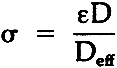where: ω

= tortuosity

ε

= porosity

D

= binary gas phase diffusivity

Deff

= effective diffusivity in porous medium.

Tortuosity represents the resistance to diffusion through a porous solid due to the influence of the pore structure. It assumes that a “tortuous path” delays the diffusion of a component compared to a “straight line” path. The concept of tortuosity is a mathematical and conceptual description that can help visualize the importance of the structure to diffusional processes. In order to find the tortuosity, the porosity had to be found. This was done by placing the weighed block of paper in a 2-liter beaker. Silicone oil was added and the beaker was put in a vacuum oven for three hours. The silicone oil replaced the air in the void spaces. The paper block was removed and weighed. From the density of the oil and the weight drawn into the block, the porosity of the paper block was found to be 0.26. Literature values for the binary gas diffusion coefficient for sulfur dioxide in air were corrected to the conditions of this experiment and used to find the tortuosity. Diffusion normal to the paper block gave a tortuosity of 4.7 whereas diffusion parallel to the paper gave a tortuosity of 1.0.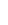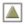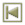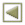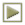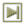Copyright � 1986 American Institute for Conservation of Historic and Artistic Works